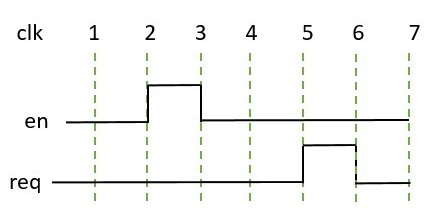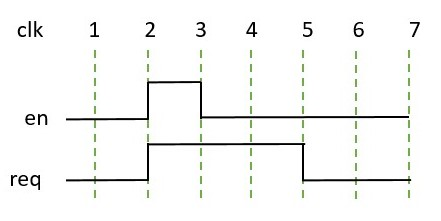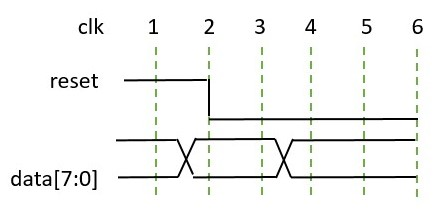Sample value functions - VLSI Verify

Tutorials

For accessing the sampling values of an expression, there are few system functions provided by SystemVerilog.

System functions can be used to access current sampled values., past sampled values, and capture changes in the sampled values.

The sample value function can be written in the assertions as well as inside procedural blocks.

## \$rose function

If there is a change in the least significant bit (LSB) of an expression from 0 or x or z (previous clocking event value) to 1, then the \$rose function returns true, otherwise returns false.

Syntax:

``\$rose (expression, <clocking_event>) ``

To understand the importance of \$rose, let’s understand how it is used for a design requirement.

### Design specification

For a request, ready should be asserted after 3 clk cycles.``````property prop;
endproperty``````

If there is a bug in RTL and ready is asserted before 3 clock cycles.

The above property will not capture this RTL defect. So, \$rose(ready) is used to capture such a bug.``````property prop;
endproperty``````

### Example in procedural block

``always @(posedge clk) out <= \$rose(ack) & valid;``

### Example for continuous assignment

``assign out = \$rose(ack, @posedge clk);``

### Difference between @posedge and \$rose

@(posedge <signal>) is true when its value changes from 0 to 1.

\$rose(<signal>) is evaluated to true for value changs happening across two clocking events from 0 or x or z to 1.

## \$fell function

If there is a change in the least significant bit (LSB) of an expression from 1 or x or z (previous clocking event value) to 0, then the \$fell function returns true, otherwise returns false.

Syntax:

``\$fell (expression, <clocking_event>)``

### Design specification

If the signal reset goes low (means reset is removed) then data should not be X or Z``````property prop;
\$fell(reset) |->  not(\$isunknown(data));
endproperty``````

### Example in procedural block

``always @(posedge clk) data = \$fell(reset)? 0: x;``

### Example for continuous assignment

``assign out = \$fell(in1 & in2, @posedge clk);``

## \$stable function

If there is no change in the value of an expression from the previous clocking event value then the \$stable function returns true, otherwise returns false.

Syntax:

``\$stable (expression, <clocking_event>)``

Example: When valid is set, associated data is expected to be true.

``````property prop;
valid |-> \$stable(wdata);
endproperty``````

### Example in procedural block

``````always @(posedge clk) begin
if(!(\$stable(data) && valid)) \$display("data is not stable when valid is high");
end``````

Note:

1. Both \$rose and \$fell function only consider the LSB bit of an expression. So, it is better to use a single-bit signal.
2. The clocking event is optional to use.
3. If clocking event is not used then
a. Derived from a clocking event used in an assertion.
b. Inferred clock from procedural block

## \$past function

The \$past() function is used to sample/ return the value of an expression or signal for the given number of clock cycles in the past.

Syntax:

``\$past(<expression>, <number_of_cycles>, <gating_expression>, <clock_event>);``
1. The default value for the number of clock cycles is 1 if not specified.
2. The gating expression is an optional expression for the clocking event.
3. A clocking event is also an optional event. It will infer an assertion or property clocking event if it is not specified.

### Design specification

For a request, ready should be asserted after 3 clock cycles. In another way, req should be set 3 clock cycles before ready is asserted.

``````property prop;
endproperty``````

### Example in procedural block

The previous cycle value of the req signal is used in the below example.

``````always @(posedge clk) begin
out <= ack & \$past(req);
end``````

## \$onehot

The \$onehot returns true if a single bit of an expression/ signal is high, otherwise for an expression/ signal has x or z value, \$onehot will fail.

Syntax:

``\$onehot(<expression>)``

### Design requirement

To read data from SRAM, there can be multiple requests from various sources. There can be only one done signal along with a ready signal.

``````property prop;
@posedge(clk) disable iff (rst) ready |-> \$onehot(done)
endproperty``````

Whenever ready is high at the same cycle, the done signal should have at least one bit to be high.

## \$onehot0

The \$onehot0 returns true if at most one bit of an expression/ signal is high (i.e. all bits are 0 or at least one bit is 1) otherwise for an expression/signal that has x or z value, \$onehot0 will fail.

Syntax:

``\$onehot0(<expression>)``

## \$isunknown

The \$isunknown returns true if any bit of an expression or signal is x or z.

Syntax:

``\$isunknown(<expression>)``

Example: When en bit is set, addr and data signal should not have x or z value.

``````property prop;
endproperty``````

## \$countones

The \$countones returns the count of ones in an expression.

Syntax:

``\$countones(<expression>)``

Example: For an error in the transaction, the data[3:0] signal value is expected to be 4‘hF. Below assertion checks all bit sets in case, an error is detected.

``````property prop;
@posedge(clk) (valid & error) |->  (\$countones(data) == 4);
endproperty``````

Note: countones do not count x or z values in an expression.

## \$assertoff(), \$asserton(), \$assertkill()

To have global control over assertions at instance or module level, \$assertoff(), \$asserton() and \$assertkill() is used.

\$assertoff() – It is used to turn off assertions temporarily at the module or instance level.

\$assertkill() – It is used to kill currently executing assertions.

\$asserton() is by default set that keeps assertion enabled by default. The \$asserton can also be called after assertoff() or \$assertkill() to enable assertion once again.

Syntax:

``````\$assertoff(level, <list of modules/ instance/ assertion_identifier>);
\$assertkill(level, <list of modules/ instance/ assertion_identifier>);
\$asserton(level, <list of modules/ instance/ assertion_identifier>);``````

where, The level represents up to what level of hierarchy from module or instance assertion can be turned on/ off.

assertion _identifier represents a label used with assert or property.

level = 0: This turns on/off assertions at all levels below the current instance or module.

….

level = n: This turn on/off assertions at ‘n’ hierarchical levels below the current instance or module.

Example: Generally assertions are turned off when reset is asserted as shown below.

``````module control_assertion();
Initial begin
@(posedge rst) \$assertoff(0, tb_top.DUT); // To disable assertion for all levels on active high reset
@(negedge rst) \$asserton(0, tb_top.DUT);  // To enable assertion for all levels on active high reset
end
endmodule``````

SystemVerilog Assertions